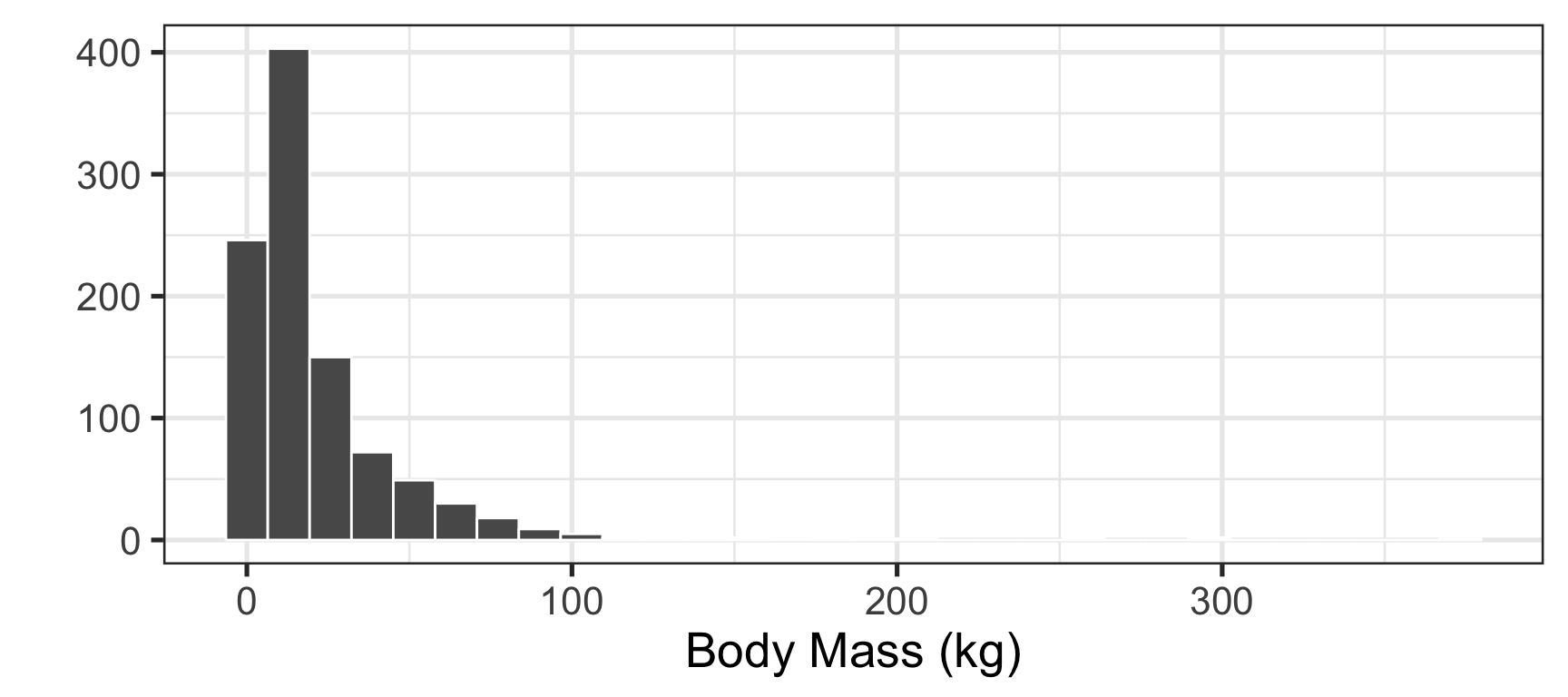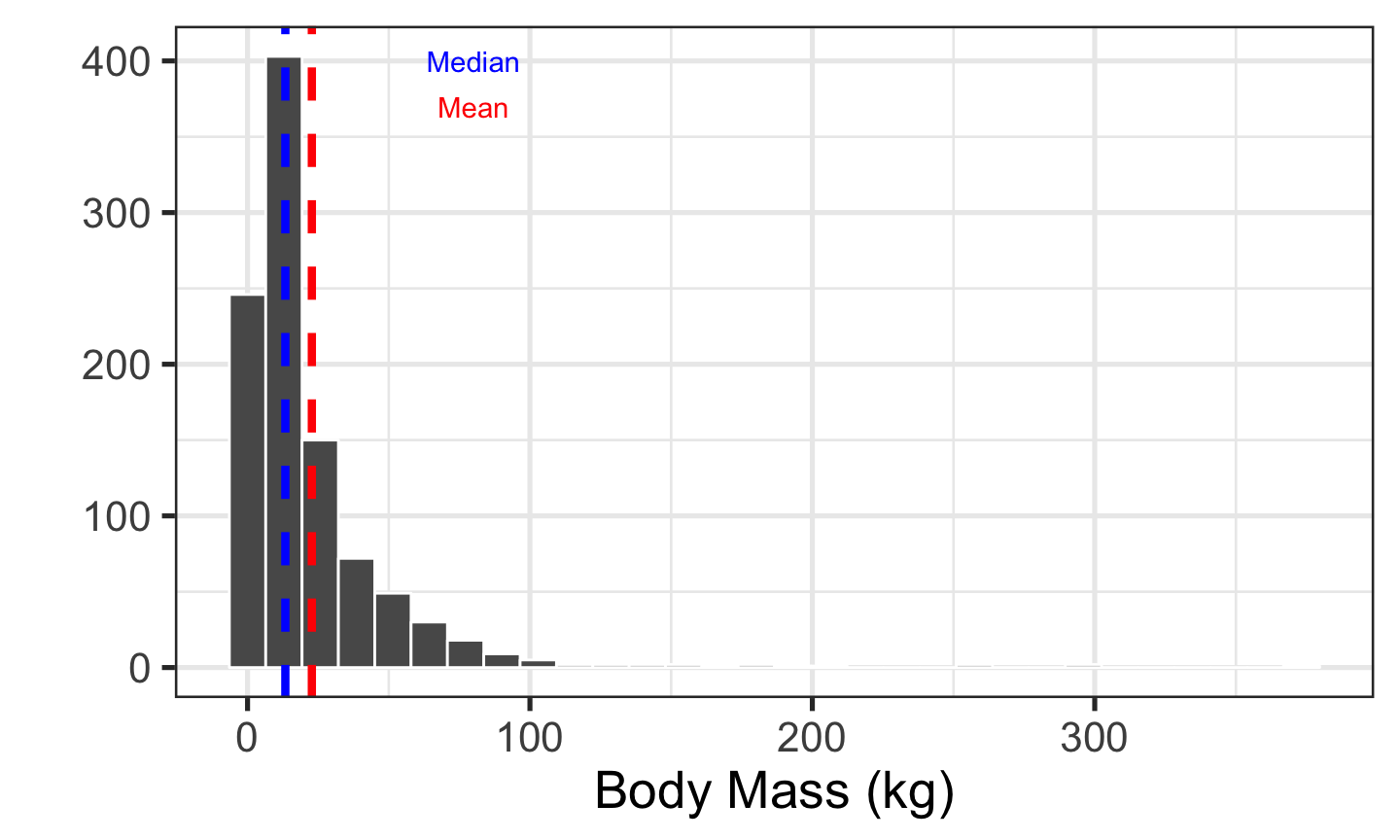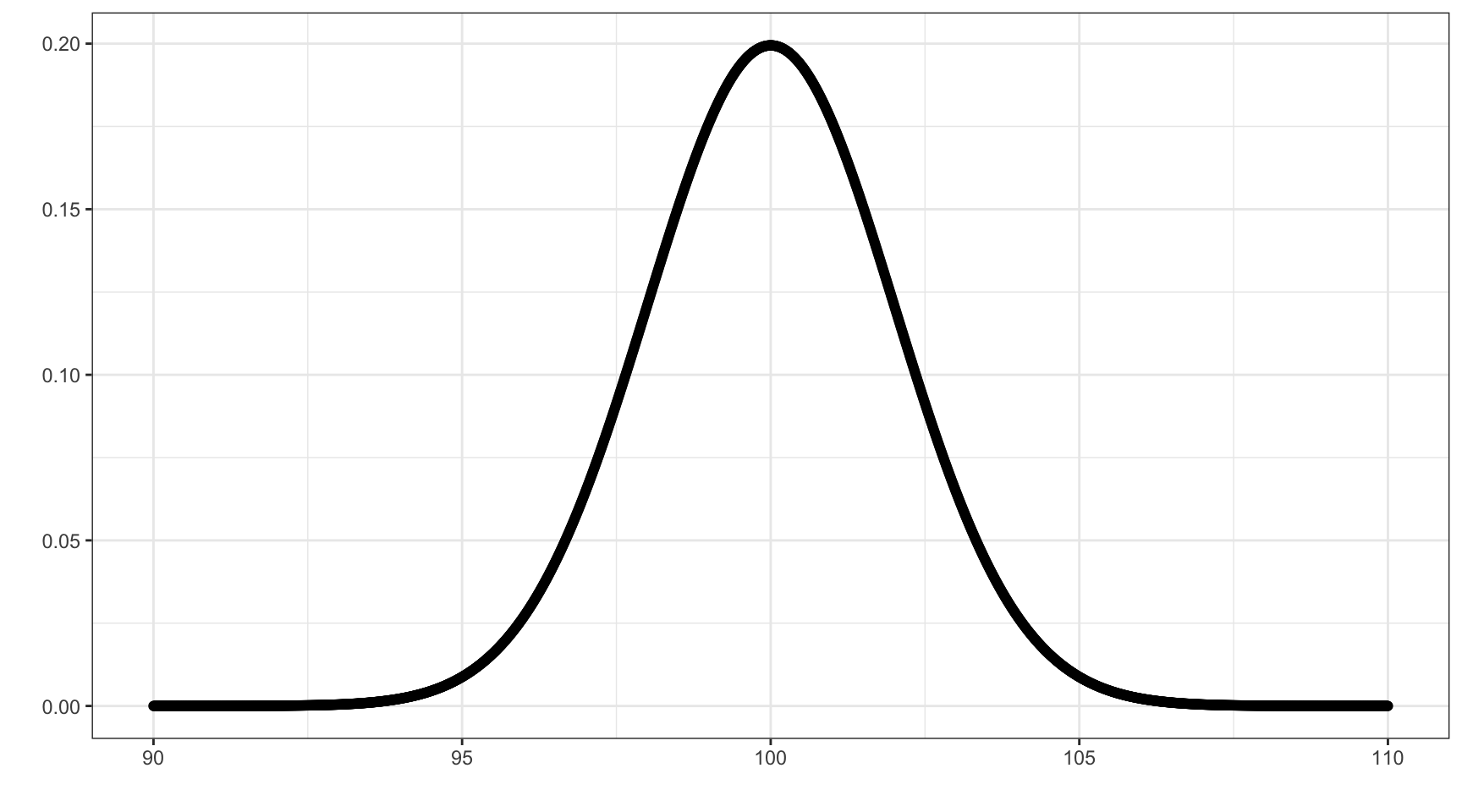## Summary Stats

Summary statistics summarize the central tendency (or location) and spread of a distribution.

library(ggplot2)
mammalmass <- rlnorm(1000, meanlog = 2.6)
bmplot <- qplot(mammalmass, xlab="Body Mass (kg)", color=I("white")) + theme_bw(20)
bmplot## Location

The most basic question to ask is where the central tendency of this distribution is. There are a variety of measures of central tendency.

## Mean

The arithmetic mean (often called the average and abbreviated $$\bar{Y}$$) is the most familiar.

$\bar{Y} = \frac{ \sum\limits_{i=1}^{n} Y_i}{n}$

where $$n$$ is the number of observations in the sample.

The mean is relatively easy to understand, but has limitations.

It is very sensitive to outliers, so the mean of the sample need not be very close to many of the actual observations.

Other measures of central tendency include the median, mode, and geometric mean.

## Median

The median is the middle value in an ordered distribution (or the average of the two middle values if there are an even number of values in the distribution).

What is the median and what is the mean?
1 , 2, 3 , 4 , 5 , 6 , 101

Median is much less sensitive to outliers than the mean.

e.g. mean income is sensitive to the super rich, and thus reflects an income that not very many people earn.

Median income reflects an income that many more people actually earn.

## Median

The plot below shows the mean versus the median for the mammalmass variable.## Mode

The mode is the peak of the probability density function (i.e., the most likely value, or most common value in a sample).

There is no really easy way to calculate the mode of a continuous variable in R, but it can be easily detected visually.

A distribution may have more than one mode.

## Mean, Median, Mode

When will these values be the same, and when different?## Geometric Mean

The geometric mean is defined as the $$nth$$ root of the product of $$n$$ numbers.

The geometric mean is especially useful for summarizing values that are of different scale.

A common use is to calculate an overall size variable from a series of measurements that have very different scales (e.g. the length of a toe bone, and the length of a femur).

Measurements of spread tell us how values are distributed around a central tendency. The most basic measure of spread is the variance:

## Variance

The variance is the mean squared deviation divided by the number of observations minus 1.

$s^2 = \frac{\sum\limits_{i=1}^n (Y_i - \bar{Y})^2}{(n-1)}$

It describes how spread out the values tend to be around the mean value.

It is also known as the mean square.

## Standard Deviation

The sample standard deviation is probably the most commonly reported measure of spread.

It refers to the square root of the variance.

Standard deviations are related to 95% confidence intervals in that 95% of the observations in a normal distribution will fall within $$\pm$$ 1.96 standard deviations.

The sample standard deviation divided by the square root of the sample size is known as the standard error of the mean, or standard error.

The standard error and standard deviation are both widely reported in the literature, and can be converted back and forth as long as the sample size is reported.

## Moments

The arithmetic mean is sometimes referred to as the first central moment of the distribution.

The variance is also called the second central moment. The third central moment indicates the skewness and the fourth central moment indicates the kurtosis (pointiness or flatness) of the distribution.

## Population versus sample

The foundation of frequentist statistics (which is most of what we will do) is that the population level parameters $$\mu$$, $$\sigma$$, and $$\sigma^2$$ have true, fixed values.

We can never know these true fixed values, so we are forced to approximate them with $$\bar{Y}$$, $$s$$ and $$s^2$$.

The Law of Large Numbers proves that if the population were sampled an infinite number of times, the average of our $$\bar{Y}$$ approximations would be identical to $$\mu$$.

This is where the name frequentist comes from…if we repeat our experiment an infinite number of times, the most frequent sample estimates of our parameters will converge on the true population value.